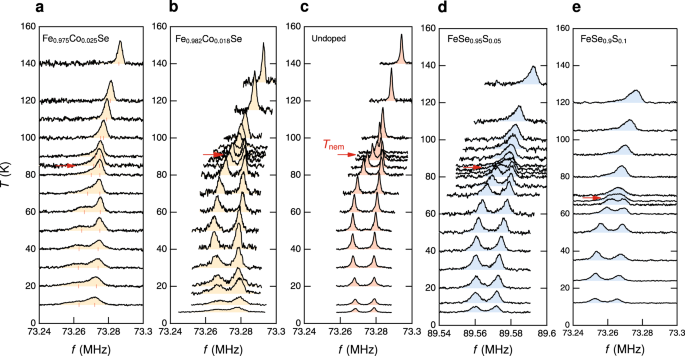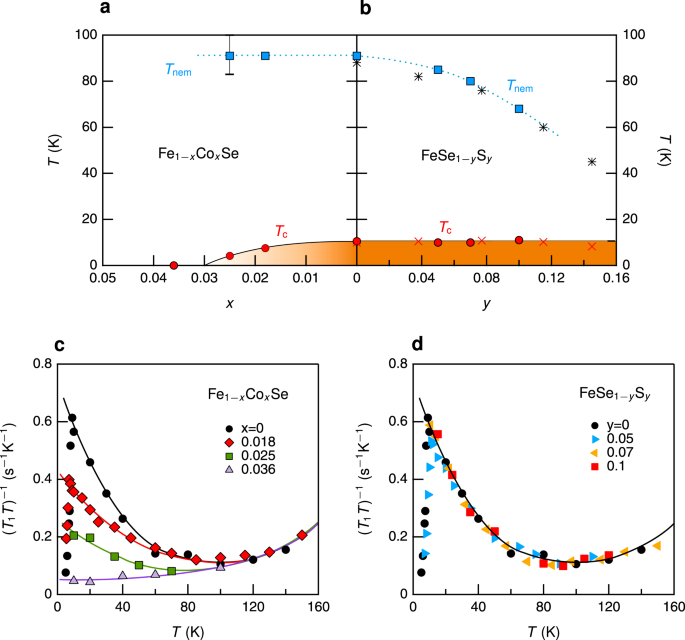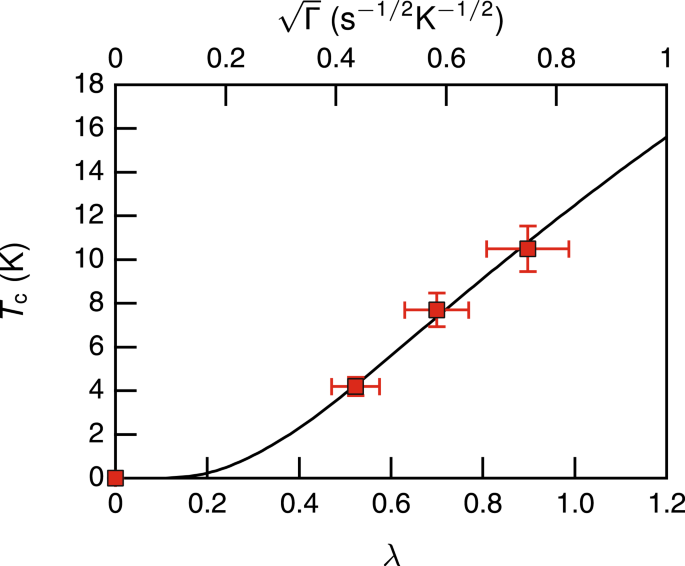## Introduction

In correlated Fermi fluids, nematicity refers to the state in which rotational symmetry is spontaneously broken, while time-reversal invariance is preserved, and consequently, the symmetry of the crystal changes from tetragonal to orthorhombic.1 An important aspect in iron-based superconductors (FeSCs) is the propensity for nematic ordering, which is usually followed by a spin-density-wave (SDW) transition, near a superconducting (SC) dome.2,3,4 Regardless of the origin of nematicity that is still under debate,5,6 this raises the fundamental issue of whether superconductivity in FeSCs is closely related to nematicity7,8 or magnetism9,10 or both.11 To address this issue, it is much desirable to separate nematic order from the magnetic one. In this respect, FeSe has been a key platform for studying the origin of nematicity and its role in superconductivity,12 as it exhibits nematic and SC orders at well-separated temperatures, Tnem ~ 90 K and Tc ~ 9 K, respectively, without involving magnetic order. Numerous recent studies in FeSe show that nematicity causes the strongly anisotropic SC gap symmetry,13,14,15,16 and further discuss that nematic fluctuations might play an important role for the superconducting pairing mechanism.17,18 On the other hand, the leading role of spin fluctuations (SFs) in the SC mechanism of FeSe, as in other FeSCs whose parent materials magnetical orders, has been also proposed in the literature.19,20,21,22 In this spin fluctuation-mediated pairing scenario, the subsequent question arises whether weak or strong coupling approach is appropriate to establish theory of superconductivity in FeSCs. It is quite interesting to note that recent NMR studies of FeSe under high pressure reveal the persistence of local nematicity at temperatures far above Tnem, which suggests a correlation between local nematicity and magnetism.23,24 Another interesting observation by NMR is the unusual suppression of $${({T}_{1}T)}^{-1}$$ at optimal pressure,25 suggesting that the interplay of SFs and superconductivity may undergo a critical change with high pressure.

As a system undergoes a nematic transition (C4C2), two nematic domains are naturally formed below Tnem, still preserving the C4 symmetry on average. Accordingly, it is usually required to detwin nematic domains, for example, by an external strain to study nematicity. As a local probe in real space, on the other hand, NMR is uniquely capable of observing the two nematic domains at the same time. Indeed, it has been established that the splitting of the NMR line in FeSCs at an external field H applied along the crystallographic a axis represents the nematic order parameter and its onset temperature corresponds to the nematic transition temperature Tnem (refs., 26,27,28 see Fig. 1c). In order to investigate whether and how nematicity is related to superconductivity, we measured the 77Se line splitting for Ha in FeSe1−ySy and Fe1−xCoxSe single crystals. In general, it is considered that substituting isovalent S for Se is equivalent to the application of (negative) chemical pressure, and Co substituted for Fe supplies an additional electron and also plays as a paramagnetic impurity. Therefore, a systematic NMR study on the two different doped systems may enable a full understanding of the relationship between nematicity, magnetism, and superconductivity.Fig. 1: 77Se NMR spectra in undoped and doped FeSe single crystals for H∥a.

## Results and discussion

Figure 1 shows how the temperature dependence of the 77Se NMR spectrum in FeSe is modified as a function of x in Fe1−xCoxSe (Fig. 1a, b) and as a function of y in FeSe1−ySy (Fig. 1d, e). For FeSe1−ySy, we find that the onset temperature of the line splitting or Tnem is gradually suppressed, consistent with previous studies.17,21 For Fe1−xCoxSe, however, Tnem hardly changes for x = 0.018. Upon further doping to slightly higher x = 0.025, the 77Se line becomes significantly broad, making it difficult to identify the onset of the line splitting. The much larger 77Se line broadening for Co doping than for S doping is well understood because Co has a strong influence on Fe moments as a nonmagnetic impurity. We notice, however, that the 77Se line broadening is not simply proportional to the concentration of Co dopants, but rather it appears to increase drastically above x ~ 0.025. In fact, for x = 0.036, it is not possible to observe the line splitting anymore, because the linewidth is much larger than the nematic splitting (see Supplementary Fig. 1). On the other hand, the 77Se linewidth is proportional to both S and Co dopants similarly, as long as Co doping is less than 2.5%, as shown in Supplementary Fig. 2. This suggests that doped Co impurities beyond ~2.5% of Fe sites cause a strong disorder effect on the correlation between Fe spins, indicating the existence of a critical doping level above which the magnetic correlation length becomes sufficiently long to induce a short-range exchange.

Although Tnem cannot be accurately determined for x = 0.025 due to the large line broadening in the nematic state, we clearly observed the line splitting below 80 K, as shown in Fig. 1a. While this puts a lower limit of Tnem, the fitting analysis of 77Se spectra in Fig. 1a also suggests that the line splitting seems to persist even up to 100 K (see vertical bars). (The detailed Knight shift data as a function of temperature and doping are shown in Supplementary Fig. 3). This suggests that Co impurities may induce a spatial distribution of Tnem in the temperature range, 80 ≤ Tnem ≤ 100 K. Regardless of the details, Tnem is marginally suppressed by Co doping within the doping range investigated, as shown in Fig. 2a. Note that the Co-doping range investigated is very narrow, and thus we are unable to argue whether Tnem remains a constant at higher Co doping. In any case, Fig. 2a, b reveals that Tnem and Tc are clearly decoupled.Fig. 2: Doping dependence of spin fluctuations for H∥a in FeSe.

Interestingly, the split 77Se lines below 80 K for x = 0.025 are notably anisotropic, i.e., the peak for the lower frequency side is broader than that for the higher frequency. The origin of the anisotropic line shape is unclear, but we note that the similar anisotropic 77Se line shape is also observed at T < 20 K for x = 0.018 (see Fig. 1b). This implies that magnetic inhomogeneity, which otherwise appears at low temperatures, prevails at higher temperatures with higher Co doping.

Contrasting sharply with the weak dependence of nematicity on both S and Co dopants, our susceptibility measurements reveal that superconductivity is strongly dependent only on Co dopants. That is, Tc is rapidly suppressed by small Co doping, whereas it is robust with regard to S doping, as shown in Fig. 2a, b, being consistent with previous studies.29,30 The very different behavior of Tnem and Tc with doping indicates that nematic and superconducting orders are not directly coupled (Coldea, A. I. et al., Evolution of the Fermi surface of the nematic superconductors FeSe1−xSx. arXiv:1611.07424, unpublished),31 raising a strong question as to whether nematicity and superconductivity are closely related.7,8,17,32

Having established the lack of a coupling of the nematic and superconducting transition temperatures, we now discuss the role of SFs for superconductivity. For probing low-energy SFs, we measured the spin-lattice relaxation rate, $${T}_{1}^{-1}$$, as the quantity $${({T}_{1}T)}^{-1}$$ is a measure of SFs at very low energy

$${({T}_{1}T)}^{-1}={\gamma }_{n}^{2}\mathop{\mathrm{lim}}\limits_{\omega \to 0}\sum _{{\bf{k}}}{A}^{2}({\bf{k}})\frac{\chi ^{\prime\prime} ({\bf{k}},\omega )}{\omega },$$
(1)

where χ″(k, ω) is the imaginary part of the dynamic susceptibility at momentum k and frequency ω, γn is the nuclear gyromagnetic ratio, and A(k) is the structure factor of the hyperfine interaction. Figure 2c, d shows $${({T}_{1}T)}^{-1}$$ as a function of Co and S doping, respectively, at Ha = 9 T. The data for the undoped FeSe crystal were taken from ref. 26 With increasing Co-doping x in Fe1−xCoxSe, $${({T}_{1}T)}^{-1}$$ or SFs above Tc is rapidly suppressed, which is exactly parallel with the suppression of Tc, as shown in Fig. 2a. Note that for x = 0.035, superconductivity is completely absent, and correspondingly SFs are not enhanced at all at low temperatures. On the other hand, $${({T}_{1}T)}^{-1}$$ above Tc is unchanged with increasing S-doping y in FeSe1−ySy up to y = 0.1, as precisely as Tc does (see Fig. 2b). From the data presented in Fig. 2, therefore, one sees that Tc depends only on the strength of spin fluctuations, but not on Tnem. (At larger S doping near y = 0.2, it was reported that both $${({T}_{1}T)}^{-1}$$ and Tc are strongly suppressed in such a way that the correlation between SFs and Tc persists,21 somewhat similar to the behavior in Co-doped samples).

For further quantitative information on how SFs are related to Tc, we adopt a spin fluctuation model in the Eliashberg formalism,33 or Millis–Monien–Pines (MMP) model.34 For this, we separate out the enhancement of $${({T}_{1}T)}^{-1}$$ that is solely associated with SFs from the data shown in Fig. 2c. Noting that $${({T}_{1}T)}^{-1}$$ for the non-superconducting sample (x = 0.035) approaches a constant, $${({T}_{1}T)}_{0}^{-1}\equiv {\Gamma }_{0}$$, without any enhancement at zero temperature, one may define the strength of SFs Γ for Ha from the $${({T}_{1}T)}^{-1}(x)$$ values just above Tc:

$$\Gamma (x)\equiv {({T}_{1}T)}^{-1}(x){| }_{{T}_{\text{c}}}-{\Gamma }_{0}.$$
(2)

While the MMP model indicates that Γ is proportional to the square of the correlation length,34 ξ2 (T), the estimation of the low- energy part of the Eliashberg bosonic spectral function suggests that the coupling constant λ is proportional to ξ, i.e., $$\sqrt{\Gamma }$$. As it was analyzed by Radtke et al.35 and Popovich et al.,36 the direct use of the MMP spectrum gives overestimation of Tc and the gap function due to a long tail at high energies of the bosonic spectral functions ~1∕ω. To cure the problem, it was proposed to introduce a cutoff or calculate the bosonic self-energy at high energies.37 For simplicity, we use the approach of cutoff proposed in ref. 36 A detailed procedure of the calculation is described in Supplementary Note 1.

The plot of Tc vs. $$\sqrt{\Gamma }$$ is shown in Fig. 3. The solid curve is a theoretical calculation (Tc vs. $$\lambda \propto \sqrt{\Gamma }$$) based on the Eliashberg theory in which electron correlation effects are substantial. The good agreement of our theory with the experimental data evidences that the magnetic scenario for superconductivity in which Cooper pairing is mediated by spin fluctuations applies to FeSe, and it is likely a universal superconducting mechanism among FeSCs.Fig. 3: Superconducting transition temperature Tc vs. $$\sqrt{\Gamma }$$, where Γ is the strength of spin fluctuations just above Tc for H∥a in Co-doped FeSe single crystals.

Based on our NMR finding that Tc relies only on SFs, the seeming relevance of nematicity with superconductivity may be simply due to the closeness with magnetism, rather than to superconductivity itself. It should be noted that the strongly anisotropic gap structure13,14,15,16,22 observed in FeSe may be a natural consequence of the presence of nematicity within the superconducting state. It is because nematicity involves the splitting of dxz and dyz orbitals that should have an inevitable influence on the gap symmetry. However, Tc itself is not necessarily affected by nematicity.38 Nevertheless, nematicity may be considered as an important barometer for superconductivity in FeSCs, as it is strongly coupled to magnetism,18 which in turn directly correlates with superconductivity.

## Methods

### Crystal growth and characterization

The growth of Fe1−xCoxSe and FeSe1−ySy single crystals was performed by using the KCl–AlCl3 flux technique in permanent T gradient in accordance with refs. 39,40 All preliminary operations for the preparation of the reaction mixture were carried out in a dry box with a residual pressure of O2 and H2O not higher than 0.1 ppm. At the first stage, polycrystalline samples of the composition Fe1−xCoxSe and FeSe1−ySy were obtained. For this, Fe, Co, S, and Se powders were carefully ground in a mortar in the appropriate ratio, and then annealed in evacuated quartz ampoules at 420 °C for a few days. In the second stage, 0.5 g of the prepared sample was placed on the bottom of a thick-walled ampoule, and then the mixture of AlCl3 and KCl in a molar ratio of AlCl3:KCl = 2:1 is added to the ampule, after that the ampule was evacuated and sealed. The sealed ampoule with polycrystalline sample of Fe–Co–Se–S loads was placed in a horizontal two-zone furnace and heated for 5 weeks in such a way that the hot-zone temperature was set to 420 °C and the cold-zone temperature was set to 370 °C. After 5 weeks, the furnace was turned off, and the ampoule was removed from the furnace. Next, the ampoule was cut, and the single crystals from the cold zone were separated from the flux by dissolving it in water. The single crystals obtained were thin square plates with metallic luster. The single crystals were grown with platelet-like morphology, and were characterized by SEM/EDX for compositional analysis.

### Nuclear magnetic resonance

77Se (nuclear spin I = 1∕2) NMR was carried out in undoped and doped FeSe single crystals at an external magnetic field, and in the range of temperature 4.2–160 K. The samples were oriented using a goniometer for the accurate alignment along the external field. The 77Se NMR spectra were acquired by a standard spin-echo technique with a typical π∕2 pulse length 2–3 μs. The nuclear spin–lattice relaxation rate $${T}_{1}^{-1}$$ was obtained by fitting the recovery of the nuclear magnetization M(t) after a saturating pulse to the following fitting function:

$$1-M(t)/M(\infty )=A\exp (-t/{T}_{1})$$

where A is a fitting parameter that is ideally unity.

### Determination of Tc and Tnem

The superconducting transition temperature Tc was determined from magnetic susceptibility (χ) measurements by comparing field-cooled and zero-field-cooled data, while we obtained the nematic transition temperature Tnem by measuring the temperature at which the 77Se NMR line splits (see Fig. 1). Due to the weakness of the signal intensity, we were unable to determine Tc by $${({T}_{1}T)}^{-1}$$ measurements, except the undoped FeSe sample. This could give an error in extracting spin fluctuations just above Tc and Γ, which was reflected in an experimental error indicated in Fig. 3.